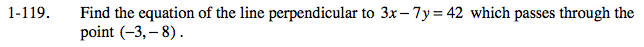### Home > PC > Chapter 1 > Lesson 1.3.2 > Problem1-119

1-119.

Find the equation of the line perpendicular to 3x − 7y = 42 which passes through the point (−3, − 8). Homework Help ✎Solve for y to find the slope.

The perpendicular slope would be the negative reciprocal of the slope of the line.

Substitute the new slope and the point into the point-slope form of a line.

$-\frac{7}{3}$